手把手教你实现神经网络

1.1 生成数据集

In :

# 导包
import matplotlib.pyplot as plt
import numpy as np
import sklearn
import sklearn.datasets
import sklearn.linear_model
import matplotlib
# 设置matplot参数
%matplotlib inline
matplotlib.rcParams['figure.figsize'] = (10.0, 8.0)

In :

# 生成数据集并用plot画出
np.random.seed(0)
X, y = sklearn.datasets.make_moons(200, noise=0.20)
plt.scatter(X[:,0], X[:,1], s=40, c=y, cmap=plt.cm.Spectral)

Out:

<matplotlib.collections.PathCollection at 0x1a1ee64f60>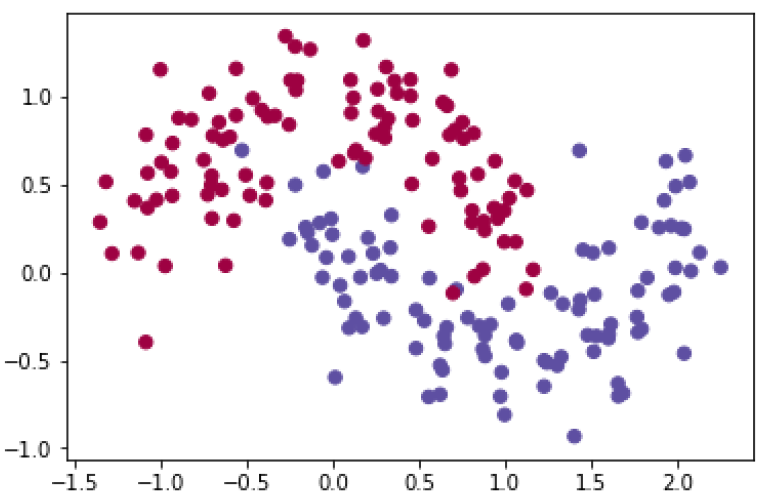1.2 逻辑回归

In :

# 训练逻辑回归分类器
clf = sklearn.linear_model.LogisticRegressionCV(cv=5)
clf.fit(X, y)

Out:

LogisticRegressionCV(Cs=10, class_weight=None, cv=5, dual=False, fit_intercept=True, intercept_scaling=1.0, max_iter=100, multi_class=’warn’, n_jobs=None, penalty=’l2′, random_state=None, refit=True, scoring=None, solver=’lbfgs’, tol=0.0001, verbose=0)

In :

# 这是个帮助函数，这个函数的作用是用来画决策边界的，如果看不懂函数内容不用介意。
def plot_decision_boundary(pred_func):
# 设置边界最大最小值
x_min, x_max = X[:, 0].min() - .5, X[:, 0].max() + .5
y_min, y_max = X[:, 1].min() - .5, X[:, 1].max() + .5
h = 0.01
# 生成一个点间网格，它们之间的距离为h
xx, yy = np.meshgrid(np.arange(x_min, x_max, h), np.arange(y_min, y_max, h))
# 预测
Z = pred_func(np.c_[xx.ravel(), yy.ravel()])
Z = Z.reshape(xx.shape)
# 绘制轮廓和训练示例
plt.contourf(xx, yy, Z, cmap=plt.cm.Spectral)
plt.scatter(X[:, 0], X[:, 1], c=y, cmap=plt.cm.Spectral)

In :

plot_decision_boundary(lambda x: clf.predict(x))
plt.title("Logistic Regression")

Out: Text(0.5, 1.0, ‘Logistic Regression’)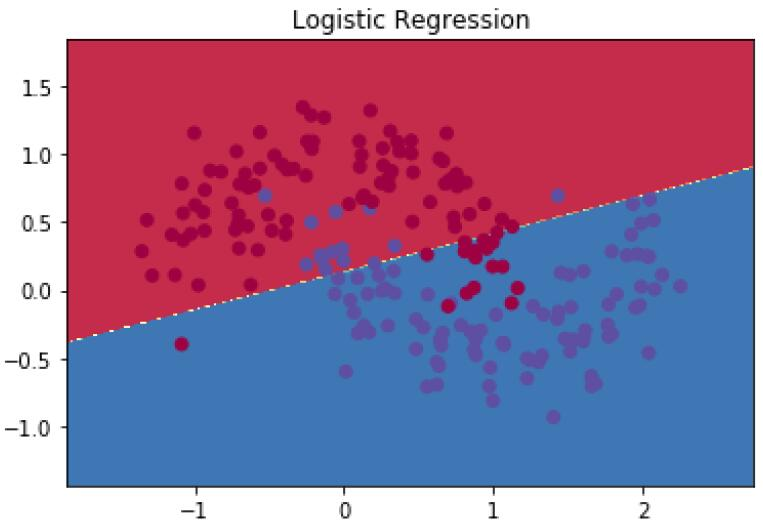1.3 训练神经网络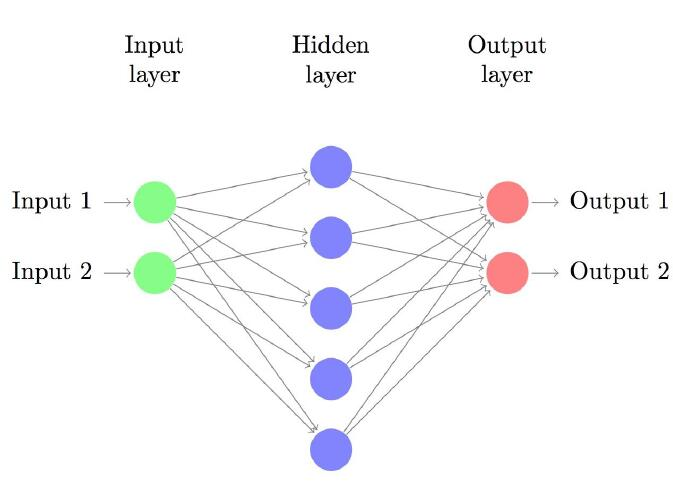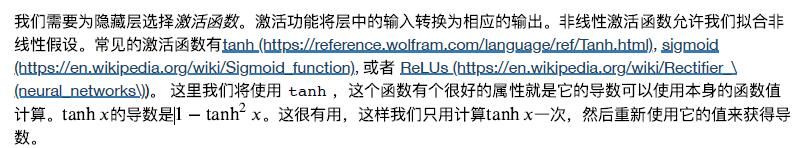1.3.2 参数学习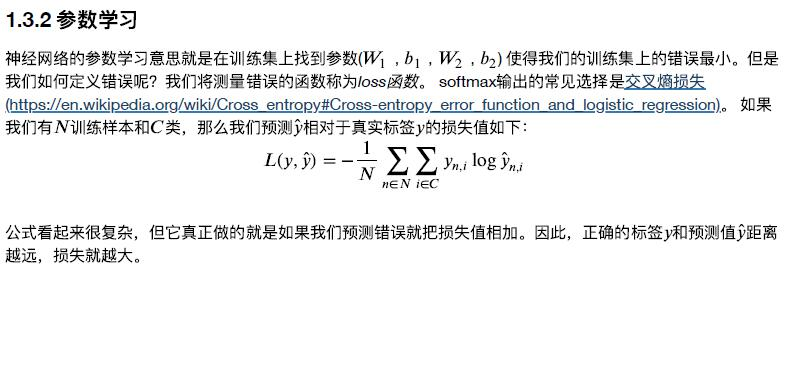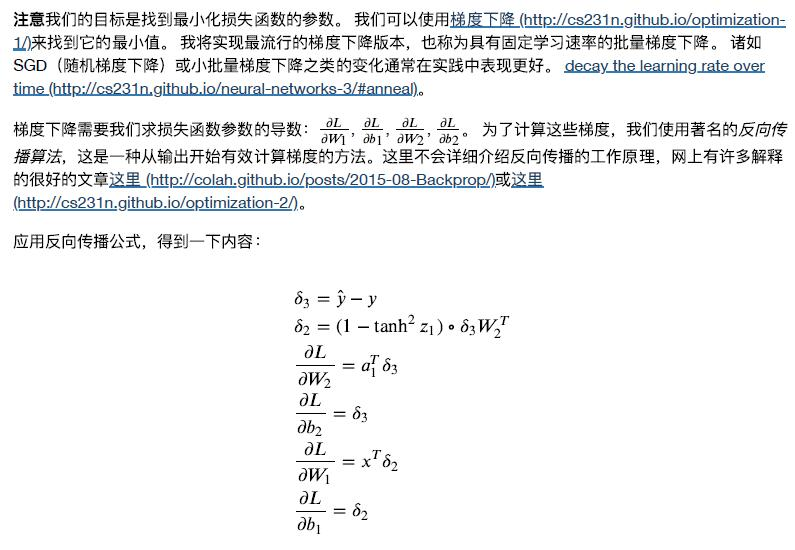1.3.3 实现代码

In :

num_examples = len(X) # 训练集大小
nn_input_dim = 2 # 输入层维度
nn_output_dim = 2 # 输出层维度
# 梯度下降参数，这两个参数是⼈为设定的超参数
epsilon = 0.01 # 梯度下降的学习率
reg_lambda = 0.01 # 正则化强度

In :

# 帮助函数用来评估数据集上的总体损失
def calculate_loss(model):
W1, b1, W2, b2 = model['W1'], model['b1'], model['W2'], model['b2']
# 前向传播来计算预测值
z1 = X.dot(W1) + b1
a1 = np.tanh(z1)
z2 = a1.dot(W2) + b2
exp_scores = np.exp(z2)
probs = exp_scores / np.sum(exp_scores, axis=1, keepdims=True)
# 计算损失值
corect_logprobs = -np.log(probs[range(num_examples), y])
data_loss = np.sum(corect_logprobs)
# 为损失添加正则化
data_loss += reg_lambda/2 * (np.sum(np.square(W1)) + np.sum(np.square(W2)))
return 1./num_examples * data_loss

In :

# 帮助函数用来预测输出类别（0或者1）
def predict(model, x):
W1, b1, W2, b2 = model['W1'], model['b1'], model['W2'], model['b2']
# 前向传播
z1 = x.dot(W1) + b1
a1 = np.tanh(z1)
z2 = a1.dot(W2) + b2
exp_scores = np.exp(z2)
probs = exp_scores / np.sum(exp_scores, axis=1, keepdims=True)
return np.argmax(probs, axis=1)

In :

# 这个函数学习神经网络的参数并返回模型。
# - nn_hdim: 隐藏层中的节点数
# - num_passes: 通过梯度下降的训练数据的次数
# - print_loss: 如果为True，则每1000次迭代打印一次损失值
def build_model(nn_hdim, num_passes=20000, print_loss=False):
# 将参数初始化为随机值。模型会学习这些参数。
np.random.seed(0)
W1 = np.random.randn(nn_input_dim, nn_hdim) / np.sqrt(nn_input_dim)
b1 = np.zeros((1, nn_hdim))
W2 = np.random.randn(nn_hdim, nn_output_dim) / np.sqrt(nn_hdim)
b2 = np.zeros((1, nn_output_dim))
# 这个是最终返回的值
model = {}
# 梯度递降
for i in range(0, num_passes):
# 前向传播
z1 = X.dot(W1) + b1
a1 = np.tanh(z1)
z2 = a1.dot(W2) + b2
exp_scores = np.exp(z2)
probs = exp_scores / np.sum(exp_scores, axis=1, keepdims=True)
# 反向传播
delta3 = probs
delta3[range(num_examples), y] -= 1
dW2 = (a1.T).dot(delta3)
db2 = np.sum(delta3, axis=0, keepdims=True)
delta2 = delta3.dot(W2.T) * (1 - np.power(a1, 2))
dW1 = np.dot(X.T, delta2)
db1 = np.sum(delta2, axis=0)
# 添加正则化项（b1和b2没有正则化项）
dW2 += reg_lambda * W2
dW1 += reg_lambda * W1
# 梯度下降参数更新
W1 += -epsilon * dW1
b1 += -epsilon * db1
W2 += -epsilon * dW2
b2 += -epsilon * db2
# 为模型分配新参数
model = {'W1': W1, 'b1': b1, 'W2': W2, 'b2': b2}
# 选择打印损失，这个操作开销很大，因为它使用整个数据集，所以不要频繁做这个操作。
if print_loss and i % 1000 == 0:
print("Loss after iteration %i: %f" % (i, calculate_loss(model)))
return model

1.3.4 隐藏层大小为3的神经网络

In :

# 隐藏层大小为3
model = build_model(3, print_loss=True)
# 绘制决策边界
plot_decision_boundary(lambda x: predict(model, x))
plt.title("Decision Boundary for hidden layer size 3")
Loss after iteration 0: 0.432387
Loss after iteration 1000: 0.068947
Loss after iteration 2000: 0.068901
Loss after iteration 3000: 0.071218
Loss after iteration 4000: 0.071253
Loss after iteration 5000: 0.071278
Loss after iteration 6000: 0.071293
Loss after iteration 7000: 0.071303
Loss after iteration 8000: 0.071308
Loss after iteration 9000: 0.071312
Loss after iteration 10000: 0.071314
Loss after iteration 11000: 0.071315
Loss after iteration 12000: 0.071315
Loss after iteration 13000: 0.071316
Loss after iteration 14000: 0.071316
Loss after iteration 15000: 0.071316
Loss after iteration 16000: 0.071316
Loss after iteration 17000: 0.071316
Loss after iteration 18000: 0.071316
Loss after iteration 19000: 0.071316

Out: Text(0.5, 1.0, ‘Decision Boundary for hidden layer size 3’)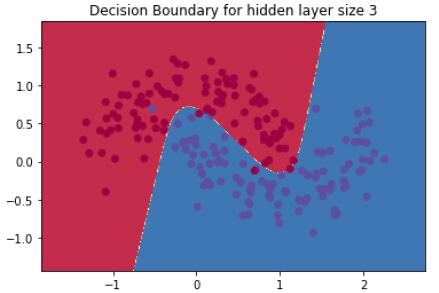2 改变隐藏的图层大小

In :

plt.figure(figsize=(16, 32))
hidden_layer_dimensions = [1, 2, 3, 4, 5, 20, 50]
for i, nn_hdim in enumerate(hidden_layer_dimensions):
plt.subplot(5, 2, i+1)
plt.title('Hidden Layer size %d' % nn_hdim)
model = build_model(nn_hdim)
plot_decision_boundary(lambda x: predict(model, x))
plt.show()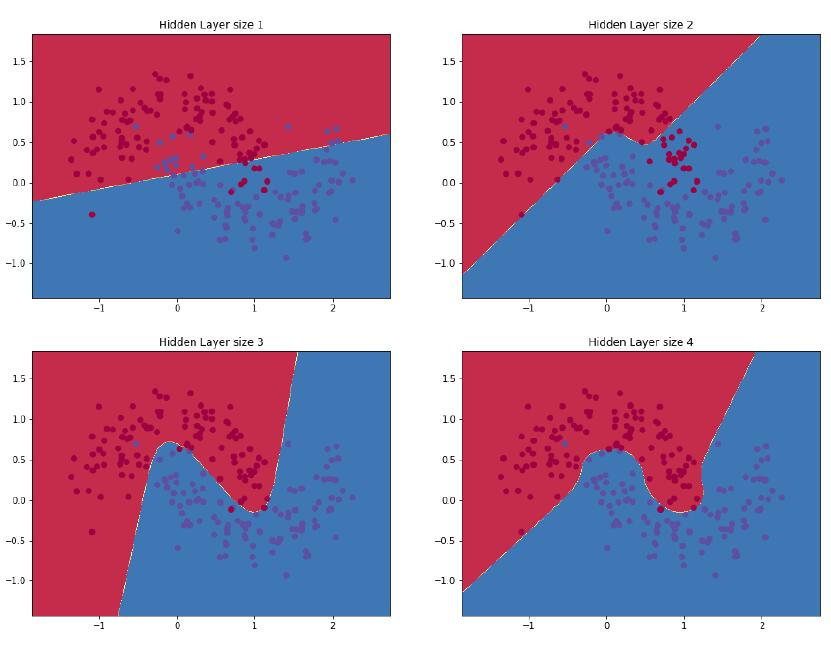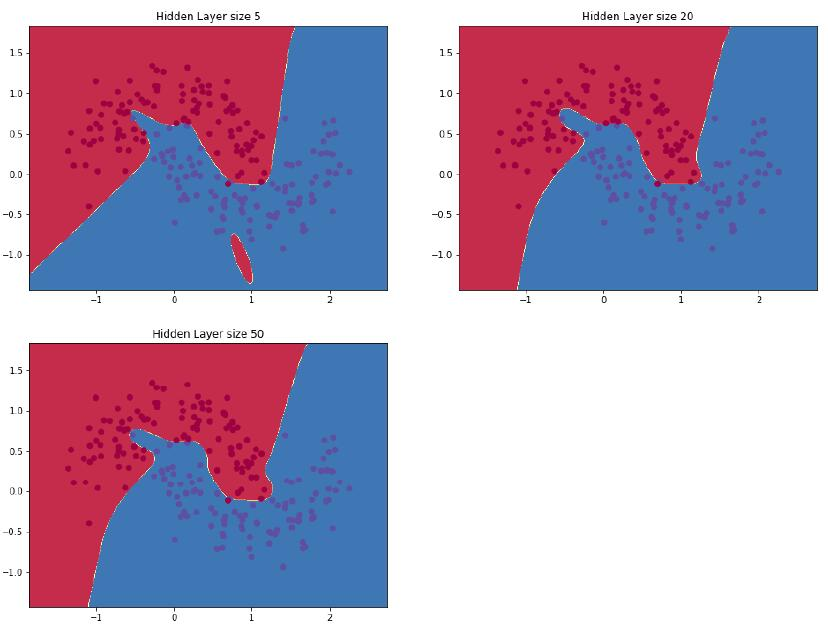Python+数据分析课程

Python教程

Python如何进行内存管理？

2021-7-11 20:38:13

Python教程

IPython是什么？怎样安装和启动IPython？

2021-7-12 2:37:59

0 条回复 A文章作者 M管理员
暂无讨论，说说你的看法吧